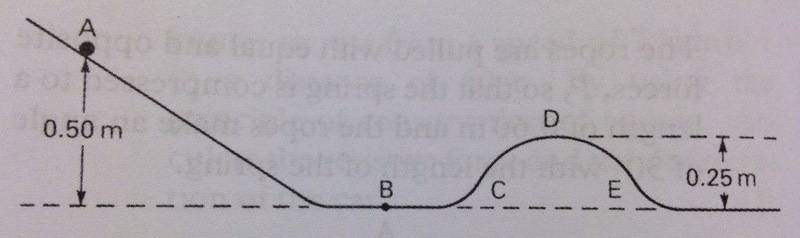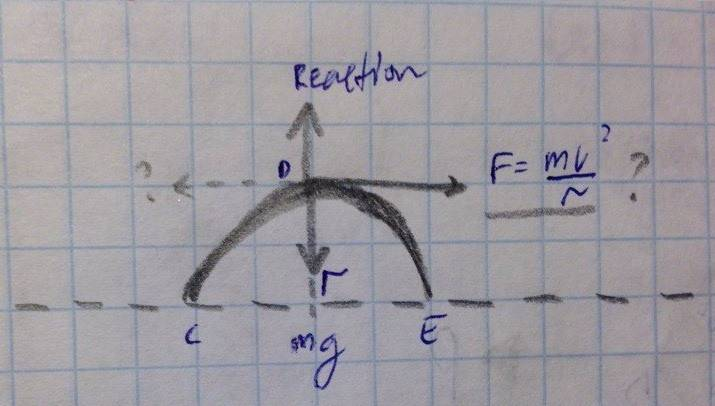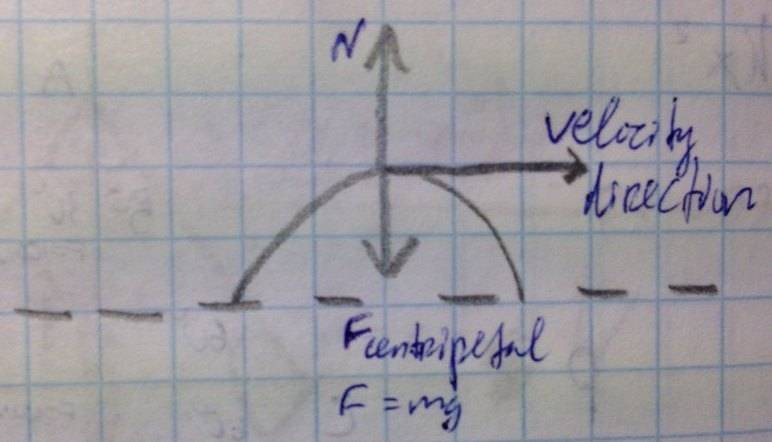# Reaction between a track and a ball at a point

## Homework Statement

The diagram shows a section of a curtain track in a vertical plane. The curved section, CDE, forms a circular arc of radius of curvature 0.75 m and the point D is 0.25 m higher than B. A ball-bearing of mass 0.060 kg is released from A, which is 0.50 m higher than B. Assume that rotational and frictional effects can be ignored and that the ball-bearing remains in contact with the track throughout the motion.

(a) Calculate the speed of the ball-bearing (i) at B, (ii) at D.
(b) Draw a diagram showing the forces acting on the ball-bearing when it is at D and calculate the reaction between the track and the ball-bearing at this point.Answers: (a) (i) 3.2 m s-1, (ii) 2.2 m s-1, (b) 0.2 N

2. The attempt at a solution
For (a) I used the following formula: v2 = u2 + 2 a s
(i) u / v0 = 0, a = 10 m s-2 (positive, since the ball is moving downwards), s = hA = 0.5 m
Plug in and get: v2 = 0 + 2 * 10 * 0.5 = 10 → v = 3.2 m s-1

(ii) u = 3.2 m s-1, a = -10 m s-2 (since the ball is moving upwards), s = h D = 0.25 m
v2 = 3.22 + 2 * (-10) * 0.25 = 5 → v = 2.2 m s-1

I think the (a) part should be correct.

In terms of (b) I am not sure. I used the F = m v2 / r formula. m = 0.060 kg, v = 2.2 m s-1, r = 0.75 m.
Plug in and get: F = (0.060 * 2.22) / 0.75 = 0.4 N, however, the answer is 0.2 N.

And this is how I see the forces acting on the ball, however, again, not sure.I have the weight (mg) and the normal reaction as the vertical forces. But regarding the horizontal ones I've got two question marks.

Any help please?

## Answers and Replies

For (a) I used the following formula: v2 = u2 + 2 a s

i would have used the energy conservation equation K.E. = massxgxH and would have got the same number for v.

In terms of (b) I am not sure. I used the F = m v2 / r formula. m = 0.060 kg, v = 2.2 m s-1, r = 0.75 m.
Plug in and get: F = (0.060 * 2.22) / 0.75 = 0.4 N, however, the answer is 0.2 N.
i do not thing F should be drawn tangential to the path and what is the argument for drawing it in this manner and then how you equate F with reaction ?

•moenste
i would have used the energy conservation equation K.E. = massxgxH and would have got the same number for v.
Thank you. Will keep that in mind. Still, I don't think that it is wrong to use v2 = u2 + 2 a s.

i do not thing F should be drawn tangential to the path and what is the argument for drawing it in this manner and then how you equate F with reaction ?
These parts I am not sure. If the ball stops at D, therefore there are no forces which influence the ball, except for the normal reaction and the weight. So, I think there are only two vertical forces and no horizontal ones.

Last edited:
Still, I don't think that it is wrong to use v2 = u2 + 2 a s.

Its definitely wrong as you are taking s to be the vertical height and a falling body will acquire a velocity in vertically downward direction and how it will turn into perpendicular direction on its own? if you use that equation then take s to be length of the incline and acceleration will havea component depending upon the angle of inclined plane.

These parts I am not sure.

Any body or particle moving on a curved path requires a centrepetal force ... and this has direction towards the centre of curvature..read motion on curved path/ banking of roads etc...
therefore your drawing of force F is incorrect..try to modify it and calculate the normal reaction which should be equal to Net down ward force.

•moenste
Its definitely wrong as you are taking s to be the vertical height and a falling body will acquire a velocity in vertically downward direction and how it will turn into perpendicular direction on its own? if you use that equation then take s to be length of the incline and acceleration will havea component depending upon the angle of inclined plane.
Thank you. KE = PE → 0.5 m v2 = m g h → v = √2 g h, I will use this formula, as you said.

Any body or particle moving on a curved path requires a centrepetal force ... and this has direction towards the centre of curvature..read motion on curved path/ banking of roads etc...
therefore your drawing of force F is incorrect..try to modify it and calculate the normal reaction which should be equal to Net down ward force.
N = [(m v2) / r] + m g = 0.4 + 0.06 * 10 = 1 NN = [(m v2) / r] + m g = 0.4 + 0.06 * 10 = 1 N

you are drawing two independent forces down ward..is it correct ? then who is or what agency is supplying the the centrepetal force?

suppose you tie a mass by string and rotate it in a vertical circle ..your fingers will pull the stone but here there is no string ,
so which force is giving/providing centrepetal force?
and if it is providing centripetal force then its own value will decrease- so net force downward will be adjusted to normal reaction between the track and the ball bearing.
if the ball was static then N=mg , but its moving on a curved path with certain speed v a part of the down ward force must be used up to sustain the motion.
your equation will have to be corrected!

•moenste
you are drawing two independent forces down ward..is it correct ? then who is or what agency is supplying the the centrepetal force?

suppose you tie a mass by string and rotate it in a vertical circle ..your fingers will pull the stone but here there is no string ,
so which force is giving/providing centrepetal force?
and if it is providing centripetal force then its own value will decrease- so net force downward will be adjusted to normal reaction between the track and the ball bearing.
if the ball was static then N=mg , but its moving on a curved path with certain speed v a part of the down ward force must be used up to sustain the motion.
your equation will have to be corrected!
Two forces are pointed downward, that's correct. Something like on this picture. Both centripetal and gravitational forces are directed downwards.

Well, if we subtract the centripetal force (0.4 N) from the weight (F = m g = 0.06 * 10 = 0.6 N) -- we will get 0.2 N (N = m g - [(m v2) / r]) -- the correct answer. However, in that case the weight should be pointed vertically upwards since the centripetal force should be directed towards the center. And I don't understand how weight or the force of gravity could be directed upwards, when it is always directed downwards (even on the picture with the buckets of water).

Update: I think this video explains this situation. We have a normal reaction directed upwards, weight directed downwards and centripetal force towards the center. Then ∑ F = m g - N = (m v2 / r) → N = m g - (m v2 / r) = 0.060 * 10 - ((0.060 * 2.22) / 0.75) = 0.2 N.

Last edited:
However, in that case the weight should be pointed vertically upwards since the centripetal force should be directed towards the center. And I don't understand how weight or the force of gravity could be directed upwards, when it is always directed downwards (even on the picture with
now you apply the newton's third law...the body is pulled by a force and the N reaction force is equal to that as there is no motion of body in vertical direction.
N- net down ward force=0
now you ask what should be net down ward force? only force available is gravitational pull but part of it must provide the centrepetal force
therefore net pull downward should be difference between the two.

suppose the velocity of the ball would be such that it could only reach the top most point. in that case the centrepetal force would use the full weight mg and the reaction will be zero.

However if you are sitting on the ball you will experience the centrifugal force(in accelerated frame) in that frame which is equal and opposite to centripetal force as you experience your weight being reduced/increased in an accelerated lift.(but this is linear motion)

•moenste
now you apply the newton's third law...the body is pulled by a force and the N reaction force is equal to that as there is no motion of body in vertical direction.
N- net down ward force=0
now you ask what should be net down ward force? only force available is gravitational pull but part of it must provide the centrepetal force
therefore net pull downward should be difference between the two.

suppose the velocity of the ball would be such that it could only reach the top most point. in that case the centrepetal force would use the full weight mg and the reaction will be zero.

However if you are sitting on the ball you will experience the centrifugal force(in accelerated frame) in that frame which is equal and opposite to centripetal force as you experience your weight being reduced/increased in an accelerated lift.(but this is linear motion)
Update: I think this video explains this situation. We have a normal reaction directed upwards, weight directed downwards and centripetal force towards the center. Then ∑ F = m g - N = (m v2 / r) → N = m g - (m v2 / r) = 0.060 * 10 - ((0.060 * 2.22) / 0.75) = 0.2 N. I think this is what you mean?

•drvrm
Update: I think this video explains this situation. We have a normal reaction directed upwards, weight directed downwards and centripetal force towards the center. Then ∑ F = m g - N = (m v2 / r) → N = m g - (m v2 / r) = 0.060 * 10 - ((0.060 * 2.22) / 0.75) = 0.2 N. I think this is what you mean?

your diagram on video is correct; and the 2nd relation is also good
N= m.g - m.V^2/r but the concept should be clear...
Perhaps you know that weightlessness in satellite is due to this feature of centripetal force being equal to grav. pull of the earth.

•moenste
your diagram on video is correct; and the 2nd relation is also good
N= m.g - m.V^2/r but the concept should be clear...
Perhaps you know that weightlessness in satellite is due to this feature of centripetal force being equal to grav. pull of the earth.
Thank you, I think I got the idea.